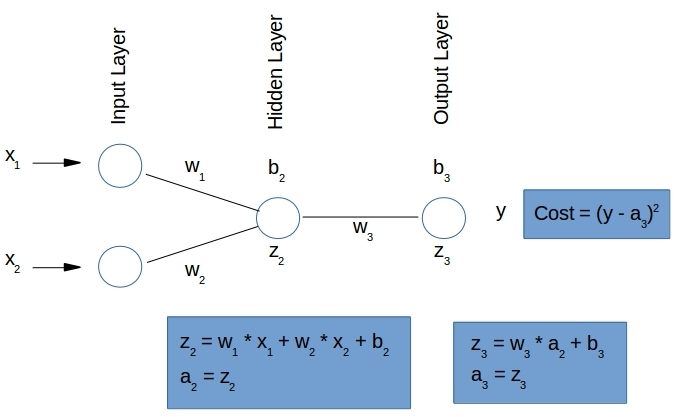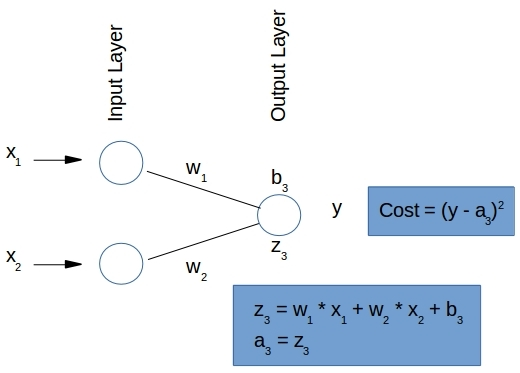# Multivariate Regression with Neural Networks: Unique, Exact and Generic Models

Michael Nielsen provides a visual demonstration in his web book Neural Networks and Deep Learning that a 1-layer deep neural network can match any function. It is just a matter of the number of neurons to get a prediction that is arbitrarily close – the more the neurons the better the approximation.  There is the Universal Approximation Theorem as well that supplies a rigorous proof of the same.But the known issues with overfitting remain and the obtained network model is only good for the range of the training data. That is, if the training data consisted only of inputs withthere would be no reason to expect the obtained network model to work outside of that range.

This series of posts are about obtaining network models that are uniquegeneric and exact. That is,

• they predict the correct output (the exact part)
• they generalize to all inputs irrespective of the data range used to train the model (the generic part)
• they can be obtained from any initial guess of the weights and biases (the unique part)

Once the training is done, the exact functional relationship between the inputs and outputs is completely captured by this neural network. That is a very desirable outcome indeed. One could consider using this neural network model as a replacement for that function in a computational framework. The function represented by the neural network model could be as simple as rotating the input vector, or perform a prescribed nonlinear transformation of the same for example. But we could envision pluggable modules of such simpler neural networks to build more complex functions.  I am not sure if there are any computational benefits to doing so at this time in the context of examples in this blog, but the possibility exists perhaps for the right applications. We will explore this in upcoming blogs in this series.

But, back to the point – are there situations where a network trained with limited data to generalize exactly for any and all input data, and converge for any initial guess?. The answer is yes.

• When there is a linear relationship between the inputs and outputs, multiple neural net models (depending on the initial guess) can make exact predictions for any input.
• Further, when we do not employ hidden layers there will be a unique model that the neural net will converge to, no matter the initial guess.

A practical (perhaps – if training the neural net is less expensive than finding the inverse of a large dense matrix) application of this is multivariate linear regression for which we have a closed form solution to compare to. If we choose the sum of squared errors as the cost function for our neural net, the model obtained should be identical to this closed form solution. We can use this known unique solution to evaluate how efficient our neural network algorithm is in converging to it from training data generated with the same model. We can study the convergence rates as a function of the learning rates, cost functions, initial guesses, the size of the training data etc… as it is neat that we have a unique solution that the network should always converge to. That is, if it is going to converge at all without running into numerical  issues owing to too large learning rates and associated numerical instabilities.

We can further look at the stability of the obtained model to noise introduced in the generated data. Covering all of this makes for a very long post, so we focus on the basics and problem formulation in this one and leave the results and implementation to a subsequent post.

## 1. The Many Degrees of Freedom

Neural networks are characterized by having a large number of parameters – the many degrees of freedom. So it is natural to expect that many combinations of these parameters can explain the outputs, given the inputs. But this does not always have to be the case. In some situations, even while we have many more parameters than constraints, there is only one possible solution to those parameters. Let us look at how this can happen in the context of neural networks.

### 1.1 Multiple exact generic models

First the case of multiple exact models all of which are generally valid. That is, irrespective of the training data range used to obtain these models, they predict the exact output for any input. Consider a simple neural net in Figure 1 that uses one hidden layer with one neuron. We want to see if we can train this neural net to add any two inputsand. What model(s) will it come up with in its attempt to minimize the error/cost is the question.Figure 1. Multiple Exact Models are possible even in the linear case when we have a hidden layer

The input to a neuron in any non-input layer is taken to be a linearly weighted function of the outputs (i.e. activations) of the neurons in the previous layer. We use identity as the activation function here, so the output of a neuron is the same as the input it gets. With the notationas the bias,the weights,the input, andthe activation – the equations shown in Figure 1 follow directly. Requiring that the output activationbe equal toi.e.we get:(1)

For Equation 1 to be true for all inputsand, we would need(2)

With 5 unknowns and 3 equations we have 2 degrees of freedom. Clearly, we are going to get multiple solutions. Choosingand(both0) as the independent variables, we get:(3)

Table 1 shows results from the neural network (Figure 1) that has been trained with identical data, but with different initial values forand. Each run drives the  cost (sum of the squared errors) to near zero, but yields a different final model. We see that the converged model in each case closely obeys Equation 3 so that the model has generic validity for any and all inputs – not just the training data range.

 b2 b3 w1 w2 w3 –b2/b3 -b3/b2 Run 1 Guess 0.04451 -0.88378 -0.86994 0.54678 0.54207 Converged 2.04097 -1.90086 1.07373 1.07373 0.93135 1.07371 0.93133 Run 2 Guess -0.52279 -1.13530 2.10237 1.42069 0.47615 Converged 4.60196 -1.90207 2.41951 2.41950 0.41332 2.41945 0.41331 Run 3 Guess 1.43401 0.62823 0.05015 -0.61086 0.62546 Converged 1.71184 1.94672 -0.87936 -0.87936 -1.13721 -0.87935 -1.13719 Table 1. Multiple Exact Generic Models. Different starting guesses for the biases and weights, converge to different models, all of which exactly predictfor anyand. The converged solutions are seen to obey Equation 3 in all cases

### 1.2 Unique exact generic model

Let us now remove the hidden layer so the neural network is as shown in Figure 2.Figure 2. Unique Exact Model. The only possible solution is obtained for any initial guess

Requiring again that the output activationbe equal towe get:(4)

The only possible solution to Equation 4 that works for allandis:(5)

This is unlike the situation when we used the hidden layer. Given that there is only one solution, the neural net has to obtain it if it is going to converge. Table 2 below bears out this result from simulating the above neural network with different initial guesses forand. We do in fact obtain the only possible solution in all cases trying to minimize the cost function.

 b3 w1 w2 Run 1 Guess -1.04436 0.00116 1.26640 Converged -1.53476e-6 0.99989 1.00003 Run 2 Guess 1.38625 -2.32841 1.24359 Converged -5.04049e-6 0.99979 1.00003 Run 3 Guess 1.04213 2.20225 -0.15782 Converged 4.10138e-7 1.00010 0.99992 Table 2. Unique Exact Generic Model. The only possible solutionandis obtained in all cases.

## 2.  Nonlinear Models

The requirement that the outputs be a linear function of the inputs for obtaining exact models is limiting. But we can accommodate the cases when the outputs can reasonably be approximated as polynomials in terms of the inputs.

### 2.1 Single input and response

A simple example is a single outputbeing a polynomial of orderin a single input.(6)

Givenmeasurements ofandwe have(7)

A least squares estimate, that minimizes, based on these measurements is known.1(8)

### 2.2 Multiple inputs and responses

Extending the above to multiple inputs and outputs (the multivariate case) is straightforward. Say we haveoutputs/responses, andactual inputs/predictors. Each measurement for a response has a form like Equation 6 but extended to include all thepredictors. It is a polynomial of degreein each predictor so we will havecoefficients in the equation. In compact matrix notation:(9)

Appealing to the single response/input case in Equations  6 and 7 it is easy to understand the following about Equation 9.

•above is simply theresponse vectors (of length, the number of measurements)  in Equation 7 stacked side-by-sde.
• Therow ofrepresents themeasurement of allresponses and thecolumn ofhas themeasurements for theresponse.
• Each column ofhas the length, the number of coefficients in the polynomial expression for the corresponding response.
•is the unit column vector. The remainingcolumns are formed from the actualpredictors contributingcolumns each. That is, each predictorcontributescolumns with values.

Given the actual measurements, the least squares estimateis similar to  Equation 8(10)

### 2.3 The neural net

Now we are ready to build a neural net that will obtain the unique exact model representing a polynomial relationship between inputs and outputs. We have to useextra inputsfor each actual input measurement, as we are targeting andegree polynomial for the outputs in each predictor variable. This is the price we have to pay in order to make the outputs a linear function of the inputs so we can use our hidden layer free neural network to obtain the unique exact model.

Having gotten all this down we will henceforth  simply use the symbolfor the number of predictors, in stead of. This is for ease of notation. The net will naturally haveinput neurons (with input),output neurons, no hidden layers, and employs linear input summation, and identity as the activation function, as shown in Figure 3.

Using the sum of squares of differences at the output layer as the cost function we have:(11)

It follows from the second derivative above that the cost function is convex infor all input data.  So we are going to march towards a model achieving the global minimum no matter what training data we use.

## 3. Conclusions

We have gone over some of the basics of the problem set up with neural networks to obtain unique, exact, and generalized target models. Building and training the network, code snippets, simulations, convergence, stability etc… will make this post too long so will be covered in an upcoming blog.

Views: 6988

Comment

Join Data Science Central